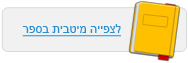עמוד:19 Activity B : Run the Energy of a Skateboard Rider simulation in slow motion ( check the box ) , lift the skateboard and rider , and then release them . Answer the following questions . Questions 1 . Match the column graphs to the illustrations . Blue indicates potential energy and yellow indicates kinetic energy . Column graph ___ corresponds to illustration A Column graph ___ corresponds to illustration B Column graph ___ corresponds to illustration C 2 . After matching the charts to the illustrations , explain how the charts describe the energy transformations in the skateboard ( note how the heights of the columns represent the amounts of each of the two types of energy ) . 3 . Choose two of the skateboarder’s positions and compare them . The criteria for comparison are the types of energy , the skater’s height above the ground , and the skater’s movement . Kinetic energy Potential energy© מטח - המרכז לטכנולוגיה חינוכית